Light It Up is a remix of the fractions game, Refraction, developed by the University of Washington's Center for Game Design. You can read a little more about the changes we've made and why here.

## The Laser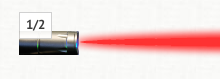The laser shoots a laser of a given fraction that can be used to light up the sensors.

The fraction that a given laser shoots is displayed on the laser, in this case the fraction is 1/2.

## The Sensor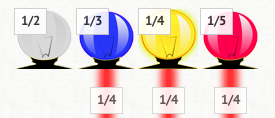The sensor will light up when a laser is directed in to the base of the sensor.

You need to make all sensors light up yellow to solve each puzzle.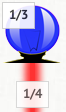#### The Blue Sensor

The sensor will turn blue if there is not enough laser being directed in to it.

In this example 1/4 is less than 1/3, so the sensor isn't getting enough laser and has turned blue.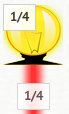#### The Yellow Sensor

The sensor will turn yellow if just the right amount of laser is being directed in to it.

In this example 1/4 is equal to 1/4, so the sensor lights up yellow.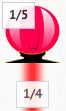#### The Red Sensor

The sensor will turn red when there is too enough laser being directed in to it.

In this example 1/4 is greater than 1/5, so the sensor is getting too much laser and has turned red.

## The Splitter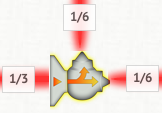The splitter will split your laser in to two lasers or three lasers.

When a splitter splits a laser, it divides that laser by two or three.

So a two-way splitter will take a 1/3 laser and produce two 1/6 lasers.

A three-way splitter will take a 1/4 laser and produce three 1/12 lasers.

1/3 ÷ 2 = 1/6 and 1/4 ÷ 3 = 1/12

## The Turner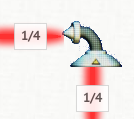The turner will redirect a laser in a new direction.

It won't change the value of the fraction on the laser at all.

## The Adder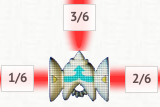The adder allows you to add together two lasers that have fractions with a common denominator.

The denominator is that number of the bottom of the fraction.

The numerator is the number on the top of the fraction.

In order to add fractions you must find a common denominator and then you simply add together the numerators.

So in order to add 1/4 + 1/8 you must convert 1/4 to 2/8 and then you can add 2/8 to 1/8 to get 3/8.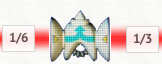If you try adding fractions without a common denominator, the adder will not produce any laser.

## Change The Denominator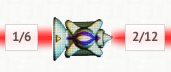This piece allows you to change the denominator of a laser's fraction, doubling it or tripling it.

When you change the denominator of a fraction you must also change the numerator that the overall value remains the same.

So if you have 1/4 and you double the denominator then you must also double the numerator, getting 2/8.

1/4 is the same as 2/8 which also equals 3/12.

In fact 1/4 = 2/8 = 3/12 = 4/16 = 5/20, etc. All of these are the same value, just with different denominators.